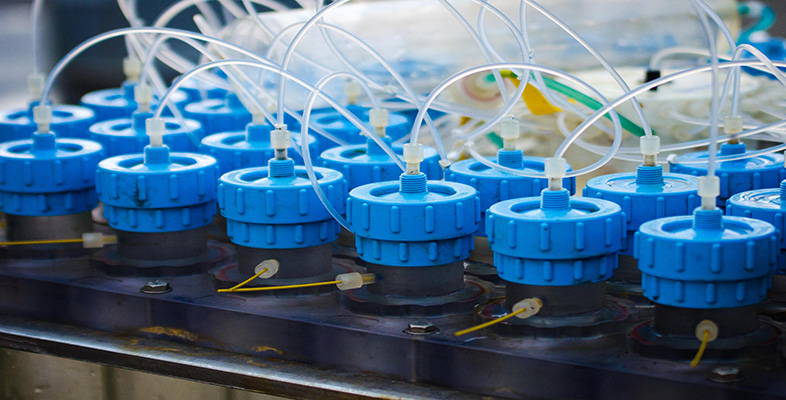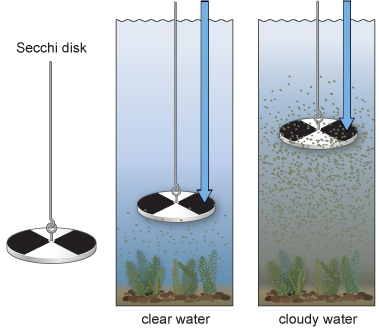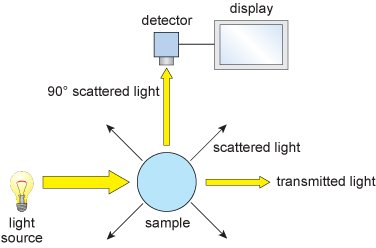Science, Maths & Technology

### Become an OU studentTest kits for water analysis

Start this free course now. Just create an account and sign in. Enrol and complete the course for a free statement of participation or digital badge if available.

# 3.2 Nitrate

Nitrate (NO3) is the most oxidised form of nitrogen, and can enter water sources from the breakdown of natural vegetation, the excess use of inorganic fertiliser and from the oxidation of nitrogen containing compounds in domestic and industrial effluents.

Many colourimetric field tests for nitrate involve azo dye formation. They are adaptations of the Griess test for nitrite, named after organic chemist Peter Griess who, in 1858, first described the reaction involved. Azo dyes are the product of the reaction between a benzene derivative (like phenol or an aromatic amine) and a diazonium salt forming an N=N bond, as shown in Equation 6. Their intense colours are exploited colourimetrically.Equation label: (6)

But to explain our analytical method, we need to take a step back and look at how the diazonium salt itself is formed, starting from nitrate ions.

The first step is to reduce nitrate (NO3) to nitrite (NO2).

To do this, most kits use cadmium metal as the reducing agent (Equation 7).

NO3 (aq) + Cd(s) + 2H+ (aq) = NO2 (aq) + Cd2+ (aq) + H2O (l)
Equation label: (7)

With cadmium, we are again working with a highly toxic substance. Although cadmium-free versions are on the market which use less toxic zinc as the reducing agent, cadmium is still the most common.

Next, in presence of an acidic buffer, nitrite is converted into nitrous acid (HNO2):

NO2 (aq) + H+ (aq) = HNO2 (aq)
Equation label: (8)

This is followed by the production of a diazonium cation as shown in Equation 6. However, in the classical Griess test, the amine in Equation 6 is replaced by sulfanilic acid (HSO3C6H4NH2(s)) to form an intermediate diazonium salt which is then combined with dihydroxybenzoic acid (also known as gentisic acid) C6H3(CO2H) (HO)2 to form a yellow-amber azo-dye (Equation 9).Equation label: (9)

The absorbance of the solution is a measure of the concentration of this compound and can be used to measure the concentration of nitrate in the original sample.

A variation on this procedure used in test strips replaces the final step above, with a coupling reaction with N-[1-napthyl] ethylenediamine to produce a red-violet azo dye.

Looking back at the initial step in the test, reduction of nitrate to nitrite, you may have spotted a problem. If nitrite ions are present in solution they would interfere with the measurement.

Nevertheless, test strip manufacturers have provided a solution.

If you go back to Figure 3, you'll see a second pad on the strip and a second reference chart on the container. This is sensitive only to nitrite; a pink-violet colouration alerts you to the presence of this ion. Nitrite can then be removed by reaction with sulfamic acid (NH2SO3H), and the measurement repeated:

MNO2 (aq) + NH2SO3H (aq) = MHSO4 (aq) + H2O(l) + N2 (g)
Equation label: (10)

where M represents a metal ion.